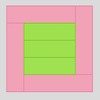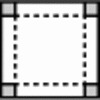#### You may also like### Cuisenaire Squares

These squares have been made from Cuisenaire rods. Can you describe the pattern? What would the next square look like?### Making Boxes

Cut differently-sized square corners from a square piece of paper to make boxes without lids. Do they all have the same volume?### A Square Deal

Complete the magic square using the numbers 1 to 25 once each. Each row, column and diagonal adds up to 65.

# Special 24

##### Age 7 to 11Challenge Level

This one is tough! Susannah from Headington Junior School has given it a try...

"The closest I can get is 8 times 8 which is 64, - 1 = 63.

63 + 63 = 126, 121 is 11 squared.

8.5 times 8.5 = 72.25, rounded up to 73.

72 + 72 = 144 which is 12 squared".

Sara from East Bergholt VC Primary, Suffolk

"At first I thought it was hard, but in the end it was easy!

840 + 1 = 841
841 = 29 x 29

840 x 2 = 1680

1680 + 1 = 1681
1681 = 41 x 41

So the Special Number is 840."